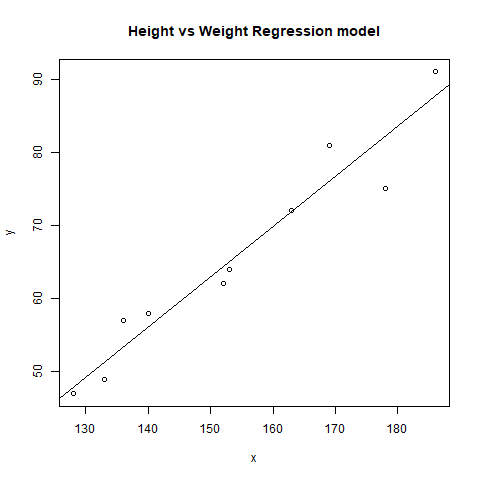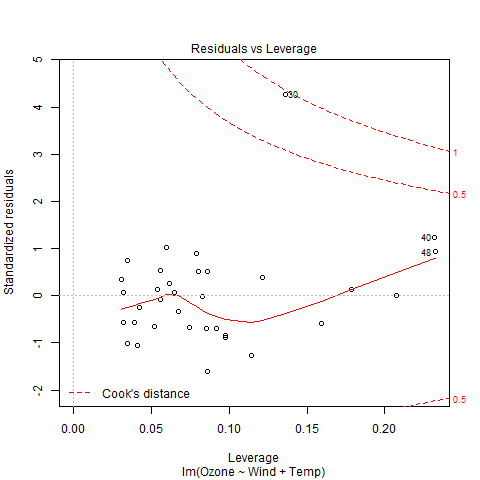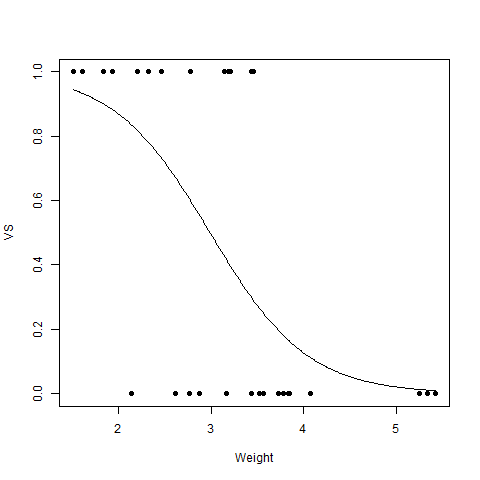# Regression and its Types in R Programming

Regression analysis is a statistical tool to estimate the relationship between two or more variables. There is always one response variable and one or more predictor variables. Regression analysis is widely used to fit the data accordingly and further, predicting the data for forecasting. It helps businesses and organizations to learn about the behavior of their product in the market using the dependent/response variable and independent/predictor variable. In this article, let us learn about different types of regression in R programming with the help of examples.

#### Types of Regression

There are mainly three types of Regression in R programming that is widely used. They are:

#### Linear Regression

Linear Regression model is one of the widely used among three of the regression types. In linear regression, the relationship is estimated between two variables i.e., one response variable and one predictor variable. Linear regression produces a straight line on the graph. Mathematically,where,
x indicates predictor or independent variable
y indicates response or dependent variable
a and b are coefficients

##### Implementation in R

In R programming, lm() function is used to create linear regression model.

Syntax:
lm(formula)

Parameter:
formula: represents the formula on which data has to be fitted
To know about more optional parameters, use below command in console: help(“lm”)

Example:
In this example, let us plot the linear regression line on the graph and predict the weight based using height.

 # R program to illustrate  # Linear Regression     # Height vector  x <- c(153, 169, 140, 186, 128,         136, 178, 163, 152, 133)     # Weight vector  y <- c(64, 81, 58, 91, 47, 57,         75, 72, 62, 49)     # Create a linear regression model  model <- lm(y~x)     # Print regression model  print(model)     # Find the weight of a person   # With height 182  df <- data.frame(x = 182)  res <-  predict(model, df)  cat("\nPredicted value of a person                  with height = 182")  print(res)     # Output to be present as PNG file  png(file = "linearRegGFG.png")     # Plot  plot(x, y, main = "Height vs Weight                   Regression model")  abline(lm(y~x))     # Save the file.  dev.off()

Output:

Call:
lm(formula = y ~ x)

Coefficients:
(Intercept)            x
-39.7137       0.6847

Predicted value of a person with height = 182
1
84.9098#### Multiple Regression

Multiple regression is another type of regression analysis technique that is an extension of the linear regression model as it uses more than one predictor variables to create the model. Mathematically,##### Implementation in R

Multiple regression in R programming uses the same lm() function to create the model.

Syntax:
lm(formula, data)

Parameters:
formula: represents the formula on which data has to be fitted
data: represents dataframe on which formula has to be applied

Example:
Let us create multiple regression model of airquality dataset present in R base package and plot the model on the graph.

 # R program to illustrate  # Multiple Linear Regression     # Using airquality dataset  input <- airquality[1:50,            c("Ozone", "Wind", "Temp")]     # Create regression model  model <- lm(Ozone~Wind + Temp,               data = input)     # Print the regression model  cat("Regression model:\n")  print(model)     # Output to be present as PNG file  png(file = "multipleRegGFG.png")     # Plot  plot(model)     # Save the file.  dev.off()

Output:

Regression model:
Call:
lm(formula = Ozone ~ Wind + Temp, data = input)

Coefficients:
(Intercept)         Wind         Temp
-58.239       -0.739        1.329#### Logistic Regression

Logistic Regression is another widely used regression analysis technique and predicts the value with a range. Moreover, it is used for predicting the values for categorical data. For example, Email is either spam or non-spam, winner or loser, male or female, etc. Mathematically,where,
y
represents response variable
z represents equation of independent variables or features

##### Implementation in R

In R programming, glm() function is used to create logistic regression model.

Syntax:
glm(formula, data, family)

Parameters:
formula: represents a formula on the basis of which model has to be fitted
data: represents dataframe on which formula has to be applied
family: represents the type of function to be used. “binomial” for logistic regression

Example:

 # R program to illustrate  # Logistic Regression     # Using mtcars dataset   # To create the logistic model  model <- glm(formula = vs ~ wt,                family = binomial,               data = mtcars)     # Creating a range of wt values  x <- seq(min(mtcars$wt),   max(mtcars$wt),                0.01)     # Predict using weight  y <- predict(model, list(wt = x),                type = "response")     # Print model  print(model)     # Output to be present as PNG file  png(file = "LogRegGFG.png")     # Plot  plot(mtcars$wt, mtcars$vs, pch = 16,       xlab = "Weight", ylab = "VS")  lines(x, y)     # Saving the file  dev.off()

Output:

Call:  glm(formula = vs ~ wt, family = binomial, data = mtcars)

Coefficients:
(Intercept)           wt
5.715       -1.911

Degrees of Freedom: 31 Total (i.e. Null);  30 Residual
Null Deviance:      43.86
Residual Deviance: 31.37        AIC: 35.37My Personal Notes arrow_drop_upCheck out this Author's contributed articles.

If you like GeeksforGeeks and would like to contribute, you can also write an article using contribute.geeksforgeeks.org or mail your article to contribute@geeksforgeeks.org. See your article appearing on the GeeksforGeeks main page and help other Geeks.

Please Improve this article if you find anything incorrect by clicking on the "Improve Article" button below.

Article Tags :

2

Please write to us at contribute@geeksforgeeks.org to report any issue with the above content.